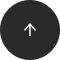Standard SQL

# How to Sum Values of a Column in SQL?

SUM

#### Problem:

You’d like to sum the values of a column.

#### Example:

Our database has a table named `game` with data in the following columns: `id`, `player`, and `score`.

idplayerscore
1John134
2Tom 146
3Lucy20
4Tom 118
5Tom 102
6Lucy90
7Lucy34
8John122

Let’s find the total score obtained by all players.

#### Solution:

```SELECT SUM(score) as sum_score
FROM game;
```

Here’s the result:

sum_score
766

#### Discussion:

The aggregate function `SUM` is ideal for computing the sum of a column’s values. This function is used in a `SELECT` statement and takes the name of the column whose values you want to sum.

If you do not specify any other columns in the `SELECT` statement, then the sum will be calculated for all records in the table. In our example, we only select the sum and no other columns. Therefore, the query in our example returns the sum all scores (766).

Of course, we can also compute the total score earned by each player by using a GROUP BY clause and selecting each player’s name from the table, alongside the sum:

#### Solution:

```SELECT player, SUM(score) as sum_score
FROM game
GROUP BY player;
```

This query returns the total score for each player:

playerscore
John256
Tom 366
Lucy144

#### Recommended courses: